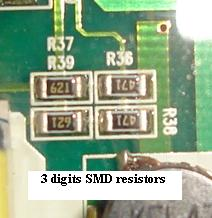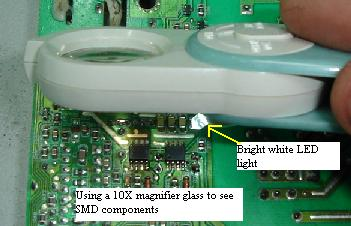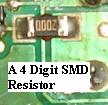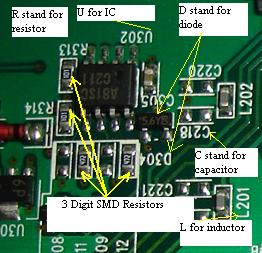## How To Calculate SMD Resistor Code FastSMD stand for surface mount device and if you repair LCD monitors, you would come across lots of SMD components. CRT monitors rarely use SMD thus it is quite easy to find out the components value. In this article, I’m going to talks about SMD resistor code and how you could calculate the values.

This SMD resistor codes is different from the normal resistor color code (color bands) you had seen in the electronic circuit boards. Surface mount resistors is very much smaller in size than the normal carbon film resistor and because of this the numbers and letters were stamped upon or printed on the top side of the component.

Those numbers and letters have a meaning and you could use that as information to find out or calculate the desire resistance values. Sometimes the resistors are designed in a very small size until we have difficulty to read the code. By using a magnifier glass we could easily read the resistor codes.There are two types of surface mount resistors used in an electronic circuit board-the 3-digit and 4-digit code SMD. The first two digits represent the two digits in the answer. The third digit represents the number of zero’s you must place after the two digits.

The answer will be in Ohms. For example: 104 which mean 10  0000 (4 zero’s) and the final answer is 100k. Let’s calculate the 4 digit SMD, 1182 which mean 118   00 (2 zero’s) and the final answer is 11.8k.

 3 Digit Example 4 Digit Example 0R1=0.1 Ohm 0000= 0 ohms= link or jumper R33=0.33 Ohm 00R1=0.1 Ohm 8R2= 8.2 Ohm 0R47=0.47 Ohm 220 is 22 Ohm and not 220 ohms 1R00=1 Ohm 331= 330 Ohms 1000= 100 Ohm 473=47000 or 47k 8202= 82000=82k

Measuring surface mount resistors is very easy with the help of digital multimeter. Most of the times when you check directly on the SMD resistors, you would get the right reading even without lifting a resistor lead. But not all digital multimeter can perform this kind of test. Select only the one that has the output voltage (measure at the probes) of less than 0.6 volts so that it won’t trigger any semiconductor devices that can give you a false reading. Once you know how to calculate the SMD resistor code and using the right way to test SMD resistors on board , you could check lots of SMD resistors in the shortest time.How to identify SMD components in circuit board